Impact Factor 3.560 | CiteScore 3.1
More on impact ›

# Frontiers in Physics## ORIGINAL RESEARCH article

Front. Phys., 27 May 2021 | https://doi.org/10.3389/fphy.2021.663573

# Wavelength Selection Method Based on Absorbance Value Optimization to Near-Infrared Spectroscopic AnalysisLijun Yao1,Xiaowen Shi1,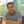Tao Pan1* andJiemei Chen2
• 1Guangdong Provincial Key Laboratory of Optical Fiber Sensing and Communications, Department of Optoelectronic Engineering, Jinan University, Guangzhou, China
• 2Department of Biological Engineering, Jinan University, Guangzhou, China

Regarding absorption spectrum, high absorption corresponds to low light transmittance and relatively loud noise, whereas low absorption corresponds to low information content, which interferes with the modeling of spectral analysis. Appropriate absorbance level is necessary to improve spectral information content and reduces noise level. In this study, based on the selection of the upper and lower bounds of absorbance, the absorbance value optimization partial least squares (AVO-PLS) method was proposed for appropriate wavelength model selection. Near-infrared spectroscopic analysis of hyperlipidemia indicators, namely, total cholesterol (TC), and triglyceride (TG), was conducted to validate the predicted performance of AVO-PLS. Well-performed wavelength selection methods, namely, moving-window PLS (MW-PLS) of continuous type-and successive projections algorithm (SPA) of discrete type, were also conducted for comparison. The spectra were first corrected using Savitzky–Golay smoothing. Modeling was performed based on the multiple partitioning of calibration and prediction sets to avoid data over-fitting and achieve parameter stability. The selected absorbance ranged from 0.45 to 0.86 for TC and from 0.45 to 0.92 for TG, and the corresponding waveband combinations were 1,376–1,388 and 1,560–1840 nm for TC and 1,376–1,390 and 1,552–1,846 nm for TG. Among them, the waveband combination of TG covers TC’s one, and can be used for the high-precision cooperativity analysis of the two indicators. Using the independent validation samples, the RMSEP and RP of 0.164 mmol l−1 and 0.990 for TC and 0.096 mmol l−1 and 0.997 for TG were obtained by the cooperativity model. And the sensitivity and specificity for hyperlipidemia were 98.0 and 100%, respectively. These values were better than those of MW-PLS and SPA. Importantly, the proposed AVO-PLS is a novel multi-band optimization approach for improving prediction performance and applicability. This method is expected to obtain more applications.

## Introduction

Near-infrared (NIR) spectroscopy achieves the rapid and simultaneous detection of multiple components of a sample. Along with the development of chemometrics, NIR spectroscopy has been successfully applied to many fields, such as soil , agricultural products and food , environment [11, 12], and biomedicine . Appropriate wavelength selection is an important but difficult aspect of using NIR spectroscopy for the reagent-free measurement of an analyte in complex samples (e.g., blood). Such selection essentially improves prediction performance, reduces complexity, and designs a specialized spectrometer with a high signal-to-noize ratio.

Moving-window partial least squares (MW-PLS) is a well-performed method for continuous wavelength selection that uses initial wavelength, number of wavelengths, and number of latent variables as the parameters to select a continuous waveband, and it has been applied to the spectroscopic analysis of many objects [35, 12, 14, 15, 19]. Other well-performed methods for discrete wavelength selection include successive projections algorithm (SPA), competitive adaptive reweighted sampling, and Monte Carlo uninformative variable elimination by PLS [710, 20]. Among these methods, SPA uses vector orthogonal projection to overcome spectral collinearity. For some analytical objects, the molecular absorption range is often a combination of multiple separate wavebands, which cannot be easily used in MW-PLS. An effective method for multi-band selection is still lacking owing to the difficulties of the algorithm.

In our previous study , an optimization algorithm was designed to determine the appropriate upper bound of absorbance and thus avoid the saturation region with high absorption. After the high absorbance wavebands were eliminated, a combination of separate wavebands was obtained and then used for further wavelength selection. The high absorption waveband with noise should be removed, and the low absorption waveband should not be used as well. Optimization of the lower bound of absorbance is also necessary because the low absorption waveband corresponded to the low information content and the noise was relatively loud. Wavelength selection could also be achieved through the selection of the upper and lower bounds of absorbance because each wavelength corresponded to an absorbance value.

In the present study, a wavelength selection algorithm called absorbance value optimization PLS (AVO-PLS) is proposed based on the selection of absorbance range. A range of absorbance values may correspond to a combination of multiple separate wavebands because different wavelengths may correspond to the same absorbance value. The AVO-PLS provides a novel approach for multi-band selection, which achieves simultaneous optimization for the lower and upper bounds of absorbance. Therefore, in terms of the algorithm, AVO-PLS is an improvement of the previous method that only avoided high absorption regions .

Total cholesterol (TC) and triglyceride (TG) are the main clinical indicators of hyperlipidemia, and they can be applied to detect cardiovascular and cerebrovascular diseases. TC and TG contain hydrogen-containing groups such as CH, CH2, and CH3, all of which have numerous absorption bands in the NIR region. A reagent-free and simultaneous analysis of TC and TG via NIR spectroscopy has been a research focus [13, 16] because it demonstrates a potential application for large-population health screening. For complex samples such as blood, using only the absorption bands of analytes is impossible because the interference of other components must be overcome. Furthermore, a combination of multiple separation bands is usually required via appropriate chemometric methods. A NIR analysis of TC and TG was conducted to validate the predicted performance of the proposed AVO-PLS. MW-PLS and SPA were also conducted for comparison. In addition, Savitzky–Golay (SG) smoothing [3, 12, 22, 23], an efficient spectral pre-processing method with a wide scope of application and different smoothing modes, was first used for the spectral pretreatment.

Modeling and parameter optimization were performed based on the multiple partitioning of calibration and prediction sets, which could effectively avoid data over-fitting and achieve parameter selection stability [3, 4, 12, 15]. The calibration, prediction, and validation processes were still performed in such an experimental design with stability.

## Materials

A total of 302 human serum samples were collected in two batches from the same hospital within two consecutive working days. The group collected on the first day (200 samples) was used for modeling, whereas the group collected on the second day (102 samples) was used for validation. Experiments were performed in compliance with the relevant laws and institutional guidelines and approved by local medical institution, which obtained the informed consent from all subjects. The TC and TG values of the samples were measured via standard clinical methods, namely, enzymatic CHOD-PAP and enzymatic GPO-PAP, respectively, using the Roche Modular PPI automatic biochemical analyzer (Roche, Switzerland) in the same hospital. All measured values ranged from 1.89 to 9.98 mmol l−1 for TC and 0.24 to 8.59 mmol l−1 for TG. The mean and SD were 4.93 and 1.08 mmol l−1 for TC and 1.34 and 0.92 mmol l−1 for TG.

In the conventional method, the phenotype-positive subjects for hyperlipidemia are those with TC > 5.20 mmol l−1 or TG > 1.70 mmol l−1 . The total samples consisted of 170 negative and 132 positive samples. The modeling group included 119 negative and 81 positive samples, while the validation group included 51 negative and 51 positive samples.

The spectroscopy instrument was an XDS Rapid Content™ Liquid Grating Spectrometer (FOSS, Denmark) equipped with a 2 mm cuvette transmission accessory. The spectra spanned 780–2,498 nm with a 2 nm interval; among them, the silicon and plumbous sulfide detections were adopted in the 780–1,100 and 1,100–2,498 nm wavebands, respectively. Each sample was measured thrice, and the mean value of the three measurements was used. The spectra were measured at 25 ± 1°C and 46 ± 1% relative humidity.

## Methods

### Evaluation Indicators in the Calibration, Prediction, and Validation Processes

The modeling set (200 samples) was further divided randomly into calibration (100 samples) and prediction (100 samples) sets 50 times. Calibration and prediction were performed for each division, and the root-mean-square error and correlation coefficient of prediction were calculated and denoted as RMSEP and RP, respectively. The mean and standard deviation of the RMSEP and RP values for all divisions were further calculated and denoted as RMSEPAve, RMSEPSD, RP,Ave, and RP,SD. The following equation,

was used as a comprehensive indicator of the modeling prediction accuracy and stability. A small RMSEP+ value indicated high model accuracy and stability. The model parameters were selected according to the minimum RMSEP+. The optimized model was then validated against the validation set (102 samples). The root-mean-square errors and the correlation coefficients of prediction in the validation set were then calculated and denoted as RMSEPV and RP,V, respectively.

In addition to the above indicators, sensitivity and specificity are direct evaluation indicators for the NIR prediction effect. The cut-off values for hyperlipidemia with the standard clinical method indicate that if the numbers of true positive, false negative, false positive, and true negative samples are a, b, c, and d, respectively, then the sensitivity and specificity of NIR analysis are calculated as follows:

Quantitative analyses of TC and TG were performed independently according to this process.

### MW-PLS

Consecutive spectral data on adjacent wavelengths were designated as a window. MW-PLS built a series of PLS models by moving window and varying window sizes, and then the optimal waveband in the spectral search region was selected according to the prediction effect. When the position and length of wavebands and the number of PLS latent variables were considered, the search parameters were set as follows: 1) initial wavelength (I), 2) number of wavelengths (N), and 3) number of PLS factors (F). The PLS model can be established for any combination of (I, N, and F) depending on the multiple partitioning of calibration and prediction sets. The corresponding RMSEPAve, RP,Ave, RMSEPSD, RP,SD, and RMSEP+ values were then calculated. The optimal waveband with minimum RMSEP+ was selected to achieve a stable and highly accurate result.

The search range included the entire scanning region (780–2,498 nm) with 860 wavelengths. The parameters I, N, and F were set to $I∈{780,782,⋯,2498}$, $N∈{1,2,⋯,860}$, and , respectively.

### SPA

SPA is an iterative forward wavelength selection method based on the absorbance matrix of the spectra of calibration samples [7, 8, 20]. Where the rows and columns of the absorbance matrix correspond to the calibration samples and spectral wavelengths, respectively, and each wavelength corresponds to an absorbance column vector.

For any fixed initial wavelength I and the number of wavelengths N, the basic algorithm of the SPA method is as follows. The initial column vector was denoted by α0. Starting from the column α0, SPA determines which of the remaining columns has the largest projection on the subspace S0 orthogonal to α0. This column, denoted by α1, can be considered as the one containing the maximum amount of information not contained in α0. In the next iteration, SPA restricts the analysis to subspace S0, considering α1 as the new reference column, and proceeds with the steps described above until a specified number N of wavelengths is reached. SPA selects wavelengths whose information content is minimally redundant so as to solve co-linearity problems.

The search parameters were described as follows: 1) initial wavelength (I), and 2) number of wavelengths (N). The search range covered the entire scanning region of 780–2,498 nm with 860 wavelengths; thus, I was set as . The maximum value of N did not exceed the number of calibration samples to avoid over-fitting. Thus, N was set as . The PLS models with the selected wavelength combination were established, and the number of PLS factors (F) ranged from 1 to 20. The optimal I, N, F were selected according to the minimum RMSEP+. The PLS models were based on several partitioning for calibration and prediction sets, which lead to stable results.

### AVO-PLS Algorithm

In the high absorption waveband, transmitted light is extremely weak and noise is relatively loud. On the contrary, in the low absorption waveband, the sample information cannot be easily detected. Wavelength selection could also be achieved through the selection of the upper and lower bounds of absorbance because each wavelength corresponded to an absorbance value. The AVO-PLS provides a novel approach for multi-band selection, which achieves simultaneous optimization for the lower and upper bounds of absorbance. In fact, Lambert-Beer law is also expressed as follows:

$T(λ)=I1(λ)I0(λ)=10−A(λ) (%),(3)$

where $λ$ is the wavelength, $A(λ)$ is the absorbance, $I0(λ)$ and $I1(λ)$ are the respective intensities of incident light and transmitted light through the sample, and $T(λ)$ is the ratio of transmitted light intensity and incident light intensity (i.e., transmittance). In the case of high absorption, for example, $A(λ)=4$, $T(λ)=0.01%$, 99.99% of the incident light was absorbed by the sample. The transmitted light was extremely weak and difficult to detect, and noise in the spectra was relatively loud. In the case of low absorption, for example, $A(λ)=0.001$, $T(λ)=99.77%$, only 0.23% of the incident light was absorbed, and the sample information almost could not be detected. Therefore, an appropriate absorbance level is necessary to improve the spectral information content and reduce the noise level.

The proposed AVO-PLS method performed the selection of appropriate upper and lower bounds of absorbance to achieve wavelength optimization. The specific procedures were as follows:

Step 1 The wavelength screening region was set as Δ, which could be the entire scanning region but could also be a portion of the region according to object and instrument properties. Meanwhile, the minimum and maximum absorbance values (Amin, Amax) were determined in the average spectrum for all samples within the wavelength screening region Δ. The increment step of absorbance was set as ε to divide the absorbance range (Amin, Amax) into n equal portions with n+1 nodes.

Step 2 Any two nodes were combined in all n+1 nodes, and the corresponding absorbance interval (A*, A*) was obtained, $\left({A}_{\ast },{A}^{\ast }\right)\subseteq \left({A}_{\mathrm{min}},{A}_{\mathrm{max}}\right)$. The relationship between wavelength and absorbance in the average spectrum indicates that a combination of wavebands that correspond to the absorbance interval (A*, A*) was selected. The obtained waveband combination was employed to establish PLS calibration and prediction models, and then the RMSEPAve, RMSEPSD, RP,Ave, RP,SD, and RMSEP+ were calculated.

Step 3 Through simultaneous traversal of A* and A*, the optimal absorbance interval (A*, A*) and the corresponding waveband combination were selected as follows:

For any fixed absorbance lower bound A*, through the traversal of A*, the local optimal absorbance interval (A*, A*), and the waveband combination were selected as follows:

For any fixed absorbance upper bound A*, through the traversal of A*, the local optimal absorbance interval (A*, A*), and the waveband combination were selected as follows:

The flow chart of the AVO-PLS algorithm is presented in Figure 1.

FIGURE 1

In this study, the wavelength screening region Δ was set as the entire scanning region (780–2,498 nm). In the average spectrum, the minimum absorbance value was greater than and close to 0, and the maximum absorbance value was less than and close to 5. Therefore, the Amin and Amax values were set as 0 and 5, respectively. The increment step of absorbance ε was set as 0.01. The absorbance range was divided into 500 equal portions by 501 nodes. The number of PLS factors (F) was set as . Figure 2 shows a sketch map of the relationship between wavelength and absorbance in the average spectrum for the case of . The corresponding waveband combination was 1,376–1,388 and 1,560–1,840 nm.

FIGURE 2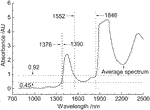FIGURE 2. Sketch map of the relationship between wavelength and absorbance in the average spectrum.

The computer algorithms for the three methods were designed using MATLAB version 7.6.

## Results

### Full Spectral Models

The NIR spectra of all 302 human serum samples in the entire scanning region (780–2,498 nm) are shown in Figure 3A. The saturation region with high absorption was mainly located near 1950 nm, whereas the low absorption region was mainly located on the left side of 900 nm. The full-PLS models based on the entire scanning region (780–2,498 nm) were established. The modeling effects (RMSEPAve, RP,Ave, RMSEPSD, RP,SD, and RMSEP+) for TC and TG are summarized in Table 1. The RP,Ave values were 0.708 and 0.864 for TC and TG, respectively, while the RMSEP+ values were 0.857 and 0.538 mmol l−1 for TC and TG, respectively. The results showed a low correlation between the NIR predicted values and the measured values of the conventional method using the spectroscopy data without pretreatment.

FIGURE 3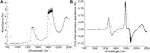FIGURE 3. NIR spectra of all human serum samples (A) raw spectra, and (B) 1st derivative spectra.

TABLE 1

The spectral data were preprocessed with SG smoothing and then the modeling was performed. The parameters of SG smoothing include order of derivatives (d), degree of polynomial (p), and number of smoothing points (m, odd). In a previous study,21 the SG mode with first-order derivative, second-degree polynomial, and 33 smoothing points (d = 1, p = 2, and m = 33) were used, and the prediction effect of PLS model for the human serum samples was improved. The SG mode (d = 1, p = 2, and m = 33) was attempted in the PLS models of TC and TG.

The corresponding first derivative spectra are shown in Figure 3B, wherein the baseline drifts of the spectra significantly decreased. The prediction effects of the corresponding PLS models with SG smoothing are also summarized in Table 1. The RP,Ave values were improved to 0.814 for TC and 0.912 for TG, while the RMSEP+ values were improved to 0.709 mmol l−1 for TC and 0.453 mmol l−1 for TG.

### MW-PLS Models

The optimal models were selected for TC and TG depending on the min RMSEP+ value using the MW-PLS method based on the SG derivative spectra. The corresponding parameters I, N, and F and the prediction effects are summarized in Table 2. The corresponding wavebands were 1,562–1,820 nm for TC and 1,538–1,836 nm for TG. Rp,Ave greatly increased to 0.988 for TC and 0.995 for TG, whereas RMSEP+ greatly decreased to 0.177 mmol l−1 for TC and 0.100 mmol l−1 for TG. The results showed that the optimal MW-PLS models with SG smoothing pretreatment were significantly better than the full-PLS models with SG smoothing pretreatment for the two indicators.

TABLE 2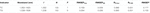TABLE 2. Parameters and modeling effects for TC and TG with MW-PLS based on SG smoothing (mmol l−1).

### SPA Models

The SPA method mentioned in SPA was employed to select the discrete wavelength combination. On the basis of the SG derivative spectra, the optimal SPA model was selected, and the corresponding I and N were 1738 nm and 56 for TC and 1,736 nm and 55 for TG, respectively. The corresponding prediction effect and parameters for the PLS models are summarized in Table 3. The results show that the SPA method was better than the full PLS method but clearly worse than the MW-PLS methods.

TABLE 3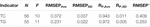TABLE 3. Parameters and modeling effects for TC and TG with SPA based on SG smoothing (mmol l−1).

### AVO-PLS Models

With the proposed AVO-PLS in AVO-PLS Algorithm, the obtained optimal absorbance intervals (A*, A*) were (0.45, 0.86) for TC and (0.45, 0.92) for TG. The transmittances ranged from 13.80 to 35.48% for TC and from 12.02 to 35.48% for TG. The corresponding waveband combinations based on the SG derivative spectra were 1,376–1,388 and 1,560–1,840 nm for TC and 1,376–1,390 and 1,552–1,846 nm for TG. TC and TG avoid extremely high or low absorption wavebands of the spectra, which correspond to a high quality of information content and a low level of noise. The parameters A*, A*, and F and the prediction effects are summarized in Table 4. The RP,Ave values were 0.990 and 0.995 for TC and TG, respectively, while the RMSEP+ values were 0.157 and 0.097 mmol l−1 for TC and TG, respectively. Tables 14 show that the optimal AVO-PLS models were significantly better than the full-PLS models and the SPA models for the two indicators, even better than the predictive effect of the optimal MW-PLS model for the two indicators.

TABLE 4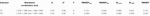TABLE 4. Parameters and modeling effects for TC and TG with AVO-PLS based on SG smoothing (mmol l−1).

It was observed that the optimal waveband combinations for TC and TG were basically the same, and the combination of TG (1,376–1,390 and 1,552–1,846 nm) completely covered the combination of TC (1,376–1,388 and 1,560–1,840 nm). Using the waveband combination of TG to analyze the indicator TC, the corresponding modeling effect was RMSEP+ = 0.158 mmol l−1 and RP, Ave = 0.988. It is very close to the effect of TC’s optimal AVO-PLS model (RMSEP+ = 0.157 mmol l−1, RP, Ave = 0.990, see also for Table 4), and there is almost no difference. Therefore, the optimal waveband combination of TG can be used for the high-precision analysis of the two indicators simultaneously.

The RMSEP+ values of the local optimal model that correspond to each fixed absorbance lower bound (A*) or upper bound (A*) are shown in Figure 4. Figures 4A,B indicated that the global optimal solution for TC was achieved at A* = 0.45, A* = 0.86, and RMSEP+ = 0.157 mmol l−1, while Figures 4C,D indicated that the global optimal solution for TG was achieved at A* = 0.45, A* = 0.92, and RMSEP+ = 0.097 mmol l−1.

FIGURE 4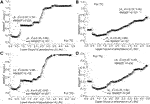FIGURE 4. RMSEP+ of the local optimal AVO-PLS model corresponding to each fixed absorbance lower bound or upper bound for (A) TC for lower bound, (B) TC for upper bound, (C) TG for lower bound, and (D) TG for upper bound.

The leftmost results in Figures 4A,C indicated that the local optimal solution for TC with fixed A* = 0.00 was reached at A* = 0.79 and RMSEP+ = 0.225 mmol l−1, while the local optimal solution for TG with fixed A* = 0.00 was reached at A* = 0.96 and RMSEP+ = 0.192 mmol l−1. These local optimal solutions corresponded to the case where only the saturation region with high absorption was eliminated. Similar works can be found in previous studies [12, 17, 21, 23]. However, compared with the global optimal solution, the predictive performance of the local optimal solution was poor. This outcome showed that only the optimization of the absorbance upper bound is insufficient.

The rightmost results in Figures 4B,D indicated that the local optimal solution for TC with fixed A* = 5.00 was reached at A* = 0.02 and RMSEP+ = 0.721 mmol l−1, whereas the local optimal solution for TG with fixed A* = 5.00 was reached at A* = 0.02 and RMSEP+ = 0.430 mmol l−1. These local optimal solutions corresponded to the case where only the low absorption region was eliminated. However, compared with the global optimal solution, the predictive performance of the local optimal solution was even poor, which showed that only the optimization of the absorbance lower bound is insufficient.

The local optimal models can also be used as valuable references. The instrument design typically involves some restrictions in the position and number of wavelengths (e.g., costs and material properties). In some instances, the demand of the actual conditions cannot be satisfied by the optimal model. Therefore, some local optimal models with prediction effects close to those of the global optimal model remain a viable option.

Figure 4A illustrates the various acceptable selections where the absorbance lower bound A* is close to 0.45, while Figure 4B shows the various acceptable selections where the absorbance upper bound A* is close to 0.86. The corresponding selections of waveband combinations were also determined easily; the modeling effects were close to the optimal model. Figures 4C,D present similar results for TG, but the relevant discussion was omitted due to the limitation in article length.

### Independence Validation

The validation group (51 negative, 51 positive, total 102), which was excluded in the modeling optimization process, was used to verify the selected SPA models (I = 1,738 nm and N = 56 for TC, and I = 1736 nm and N = 55 for TG), the selected MW-PLS models (1,562–1820 nm for TC and 1,538–1836 nm for TG) and the selected cooperativity model (1,376–1,390 and 1,552–1,846 nm for TC and TG) with AVO-PLS on the basis of the SG derivative spectra. The PLS regression coefficients were determined using the SG derivative spectra and measured reference values of the modeling samples depending on the corresponding parameters. The predicted TC and TG values were then calculated using the SG derivative spectra of the validation samples and the obtained PLS regression coefficients.

The obtained RMSEPV and RP,V values of SPA for validation were 0.386 mmol l−1 and 0.943 for TC and 0.285 mmol l−1 and 0.970 for TG. The obtained RMSEPV and RP,V values of MW-PLS for validation were 0.169 mmol l−1 and 0.989 for TC and 0.099 mmol l−1 and 0.996 for TG. The RMSEPV and RP,V of AVO-PLS were 0.164 mmol l−1 and 0.990 for TC and 0.096 mmol l−1 and 0.997 for TG, respectively. Figure 5 shows the relationship between the NIR predicted values and the measured reference values of the validation samples with the optimal MW-PLS, SPA, and AVO-PLS models for TC and TG, respectively. The three methods for the two indicators demonstrated acceptable prediction accuracy and high correlation for the clinically measured values. The prediction effects of AVO-PLS were the best on the validations of TC and TG.

FIGURE 5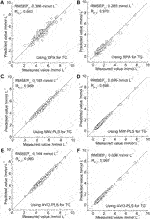FIGURE 5. Relationship between the predicted and measured values of validation samples for (A) TC with SPA, (B) TG with SPA, (C) TC with MW-PLS, (D) TG with MW-PLS, (E) TC with AVO-PLS, and (F) TG with AVO-PLS.

The prediction effect of NIR analysis was then evaluated from the criteria of sensitivity and specificity. Using SPA, the numbers of true positive (a), false negative (b), false positive (c), and true negative (d) samples are 45, 6, 3, and 48, respectively; the sensitivity and specificity were 88.2 and 94.1%, respectively. With MW-PLS, the sensitivity and specificity were 96.1 and 100% (a = 49, b = 2, c = 0, d = 51), respectively. With AVO-PLS, the sensitivity and specificity were 98.0 and 100% (a = 50, b = 1, c = 0, d = 51), respectively. Therefore, based on the evaluation criteria with sensitivity and specificity, AVO-PLS and MW-PLS are similar, both very good, and SPA is the worst. Furthermore, for AVO-PLS and MW-PLS, the classification between negative and positive for hyperlipidemia can be observed in the 2D diagram (TC and TG) with the cut-off lines (TC = 5.20; TG = 1.70). Figure 6 shows the 2D diagram of NIR predicted values of the 102 validation samples classified as negative and positive for hyperlipidemia using the two methods. The results confirmed the feasibility of hyperlipidemia screening with NIR spectroscopy.

FIGURE 6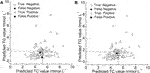FIGURE 6. 2D diagram of NIR predicted values (TC, TG) of the validatSion samples classified as negative and positive for hyperlipidemia with (A) MW-PLS and (B) AVO-PLS.

Through NIR analysis of TC and TG, AVO-PLS achieved high-precision prediction, even slightly better than the well-perfomed MW-PLS method. Unlike other single-band screening methods, such as MW-PLS, AVO-PLS can be used to select a multi-band combination, a function that is significant in physics and optics. Therefore, AVO-PLS can improve the applicability of spectral analysis.

The high water content of the serum samples can lead to saturated absorption and noise interference. The proposed AVO-PLS can reasonably eliminate the high absorbance wavebands (the upper bound of absorbance). TC and TG are lipid compounds. The results show that the predicted effects of TC and TG are not affected and evidently improved after eliminating the saturated absorption bands of water. If the water content of samples was measured, then a shorter optical path length could be used to avoid saturated absorption. In this case, the AVO-PLS can still reasonably eliminate the weak absorbance wavebands (the lower bound of absorbance). It is meaningful that the cooperativity model can detect two indicators at the same time. This provides a more concise scheme for the designing splitting systems for spectroscopic instruments.

## Conclusion

Wavelength selection is one of the difficulties of spectral analysis, especially for complex samples. Effective multi-band selection methods are still few because of the difficulty of the algorithm.

In the high absorption waveband, transmitted light is extremely weak and noise is relatively loud. On the contrary, in the low absorption waveband, the sample information cannot be easily detected. An appropriate absorbance level can improve the spectral information content and reduce the noise level, especially in the transmission spectra of liquid samples. A multi-band selection method (i.e., AVO-PLS) based on the selection of the upper and lower bounds of absorbance was proposed in this study.

NIR analysis of total cholesterol and triglycerides in human serum samples verified the effectiveness of AVO-PLS. The RMSEPV and RP,V were 0.164 mmol l−1 and 0.990 for TC and 0.096 mmol l−1 and 0.997 for TG, respectively. The AVO-PLS method achieved a high-precision prediction, which is better than the well-performed MW-PLS method. And it is meaningful that the optimal waveband combination (1,376–1,390 and 1,552–1,846 nm) of TG can be used for the high-precision cooperativity analysis of the two indicators. This provides a more concise designing for the splitting systems of spectroscopic instruments.

It is worthwhile to believe that AVO-PLS method based on the optimization of the upper and lower bounds of absorbance is an advancement in optics and spectroscopy. It implemented multi-band optimization to improve its prediction performance and applicability, and is expected to be applied to a wider field of analysis.

## Data Availability Statement

The raw data supporting the conclusions of this article will be made available by the authors, without undue reservation.

## Author Contributions

TP, LY, XS, and JC contributed to conception and design of the study. XS, LY, and JC organized the database. LY, XS, and TP performed the statistical analysis. LY, TP, and XS wrote the first draft of the manuscript. TP, LY, XS, and JC wrote sections of the manuscript. All authors contributed to manuscript revision, read, and approved the submitted version.

## Funding

This work was supported by the National Natural Science Foundation of China (No. 61078040), and the Science and Technology Project of Guangdong Province of China (No.2014A020213016, No.2014A020212445).

## Conflict of Interest

The authors declare that the research was conducted in the absence of any commercial or financial relationships that could be construed as a potential conflict of interest.

## References

1. Rossel RAV, Walvoort DJJ, McBratney AB, Janik LJ, Skjemstad JO. Visible, Near Infrared, Mid Infrared or Combined Diffuse Reflectance Spectroscopy for Simultaneous Assessment of Various Soil Properties and Assessment of Soil Spatial Variation. Geoderma (2006) 131:59–75. doi:10.1016/j.geoderma.2005.03.007

2. Morón A, Cozzolino D. Application of Near Infrared Reflectance Spectroscopy for the Analysis of Organic C, Total N and pH in Soils of Uruguay. J Near Infrared Spectrosc (2002) 10:215–21. doi:10.1016/j.tetlet.2007.06.00710.1255/jnirs.338

3. Chen H, Pan T, Chen J, Lu Q. Waveband Selection for NIR Spectroscopy Analysis of Soil Organic Matter Based on SG Smoothing and MWPLS Methods. Chemometrics Intell Lab Syst (2011) 107:139–46. doi:10.1016/j.chemolab.2011.02.008

4. Pan T, Li M, Chen J. Selection Method of Quasi-Continuous Wavelength Combination with Applications to the Near-Infrared Spectroscopic Analysis of Soil Organic Matter. Appl Spectrosc (2014) 68:263–71. doi:10.1366/13-07088

5. Pan T, Han Y, Chen J, Yao L, Xie J. Optimal Partner Wavelength Combination Method with Application to Near-Infrared Spectroscopic Analysis. Chemometrics Intell Lab Syst (2016) 156:217–23. doi:10.1016/j.chemolab.2016.05.022

6. Chen JY, Zhang H, Matsunaga R. Rapid Determination of the Main Organic Acid Composition of Raw Japanese Apricot Fruit Juices Using Near-Infrared Spectroscopy. J Agric Food Chem (2006) 54:9652–7. doi:10.1021/jf061461s

7. Galvão RKH, Araújo MCU, Fragoso WD, Silva EC, José GE, Soares SFC, et al. A Variable Elimination Method to Improve the Parsimony of MLR Models Using the Successive Projections Algorithm. Chemometrics Intell Lab Syst (2008) 92:83–91. doi:10.1016/j.chemolab.2007.12.004

8. Moreira EDT, Pontes MJC, Galvão RKH, Araújo MCU. Near Infrared Reflectance Spectrometry Classification of Cigarettes Using the Successive Projections Algorithm for Variable Selection. Talanta (2009) 79:1260–4. doi:10.1016/j.talanta.2009.05.031

9. Li H, Liang Y, Xu Q, Cao D. Key Wavelengths Screening Using Competitive Adaptive Reweighted Sampling Method for Multivariate Calibration. Analytica Chim Acta (2009) 648:77–84. doi:10.1016/j.aca.2009.06.046

10. Cai W, Li Y, Shao X. A Variable Selection Method Based on Uninformative Variable Elimination for Multivariate Calibration of Near-Infrared Spectra. Chemometrics Intell Lab Syst (2008) 90:188–94. doi:10.1016/j.chemolab.2007.10.001

11. Sousa AC, Lucio MMLM, Neto OFB, Marcone GPS, Pereira AFC, Dantas EO, et al. A Method for Determination of COD in a Domestic Wastewater Treatment Plant by Using Near-Infrared Reflectance Spectrometry of Seston. Analytica Chim Acta (2007) 588:231–6. doi:10.1016/j.aca.2007.02.022

12. Pan T, Chen Z, Chen J, Liu Z. Near-Infrared Spectroscopy with Waveband Selection Stability for the Determination of COD in Sugar Refinery Wastewater. Anal Methods (2012) 4:1046–52. doi:10.1039/C2AY05856A

13. Heise HM, Bittner A, Marbach R. Clinical Chemistry and Near Infrared Spectroscopy: Technology for Non-invasive Glucose Monitoring. J Near Infrared Spectrosc (1998) 6:349–59. doi:10.1255/jnirs.156

14. Kasemsumran S, Du Y-p., Murayama K, Huehne M, Ozaki Y. Simultaneous Determination of Human Serum Albumin, γ-globulin, and Glucose in a Phosphate Buffer Solution by Near-Infrared Spectroscopy with Moving Window Partial Least-Squares Regression. Analyst (2003) 128:1471–7. doi:10.1039/B307294K

15. Pan T, Liu J, Chen J, Zhang G, Zhao Y. Rapid Determination of Preliminary Thalassaemia Screening Indicators Based on Near-Infrared Spectroscopy with Wavelength Selection Stability. Anal Methods (2013) 5:4355–62. doi:10.1039/C3AY40732B

16. Garcoa-Garcoa JL, Pérez-Guaita D, Ventura-Gayete J, Garrigues S, Guardia MDL. Determination of Biochemical Parameters in Human Serum by Near-Infrared Spectroscopy. Anal Methods (2014) 6:3982–9. doi:10.1039/C3AY42198H

17. Han Y, Chen J, Pan T, Liu G. Determination of Glycated Hemoglobin Using Near-Infrared Spectroscopy Combined with Equidistant Combination Partial Least Squares. Chemometrics Intell Lab Syst (2015) 145:84–92. doi:10.1016/j.chemolab.2015.04.015

18. Yao L, Tang Y, Yin Z, Pan T, Chen J. Repetition Rate Priority Combination Method Based on Equidistant Wavelengths Screening with Application to NIR Analysis of Serum Albumin. Chemometrics Intell Lab Syst (2017) 162:191–6. doi:10.1016/j.chemolab.2017.01.017

19. Long X, Liu G, Pan T, Chen J. Waveband Selection of Reagent-free Determination for Thalassemia Screening Indicators Using Fourier Transform Infrared Spectroscopy with Attenuated Total Reflection. J Biomed Opt (2014) 19:087004–11. doi:10.1117/1.JBO.19.8.087004

20. Araújo MCU, Saldanha TCB, Galvão RKH, Yoneyama T, Chame HC, Visani V. The Successive Projections Algorithm for Variable Selection in Spectroscopic Multicomponent Analysis. Chemometrics Intell Lab Syst (2001) 57:65–73. doi:10.1016/S0169-7439(01)00119-8

21. Chen J, Ai T, Pan T, Yao L, Xia F. AO-MW-PLS Method Applied to Rapid Quantification of Teicoplanin with Near-Infrared Spectroscopy. J Innov Opt Health Sci (2017) 10(13):1650029. doi:10.1142/S1793545816500292

22. Savitzky A, Golay MJE. Smoothing and Differentiation of Data by Simplified Least Squares Procedures. Anal Chem (1964) 36:1627–39. doi:10.1021/ac60214a047

23. Xie J, Pan T, Chen J-M, Chen H-Z, Ren X-H. Joint Optimization of Savitzky-Golay Smoothing Models and Partial Least Squares Factors for Near-Infrared Spectroscopic Analysis of Serum Glucose. Chin J Anal Chem (Chinese Version) (2010) 38:342–6. doi:10.3724/SP.J.1096.2010.00342

24.The China Formulating Committee for Guidelines on Prevention. Treatment of blood lipid abnormality in Chinese adults. (2007). Chin J. Cardiol. 35, 390–419. doi:10.3760/j.issn:0253-3758.2007.05.003

Keywords: near-infrared spectroscopic analysis, absorbance value optimization, multi-band optimization, total cholesterol, triglycerides

Citation: Yao L, Shi X, Pan T and Chen J (2021) Wavelength Selection Method Based on Absorbance Value Optimization to Near-Infrared Spectroscopic Analysis. Front. Phys. 9:663573. doi: 10.3389/fphy.2021.663573

Received: 03 February 2021; Accepted: 05 May 2021;
Published: 27 May 2021.

Edited by:

Wei Ren, The Chinese University of Hong Kong, China

Reviewed by:

Ilia L. Rasskazov, University of Rochester, United States
Liuhao Ma, Wuhan University of Technology, China

Copyright © 2021 Yao, Shi, Pan and Chen. This is an open-access article distributed under the terms of the Creative Commons Attribution License (CC BY). The use, distribution or reproduction in other forums is permitted, provided the original author(s) and the copyright owner(s) are credited and that the original publication in this journal is cited, in accordance with accepted academic practice. No use, distribution or reproduction is permitted which does not comply with these terms.

*Correspondence: Tao Pan, 466945939@qq.com

These authors have contributed equally to this work and share first authorship